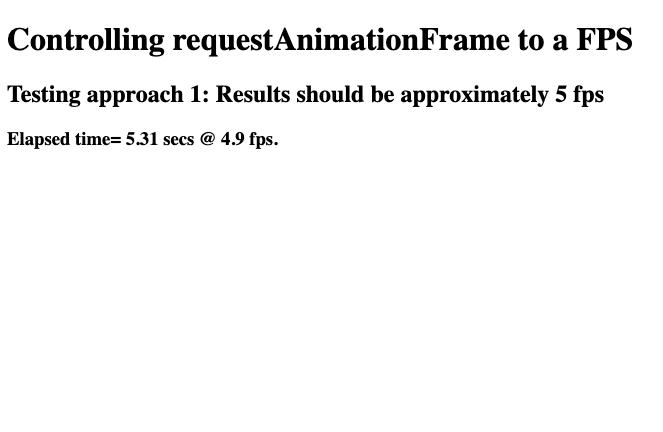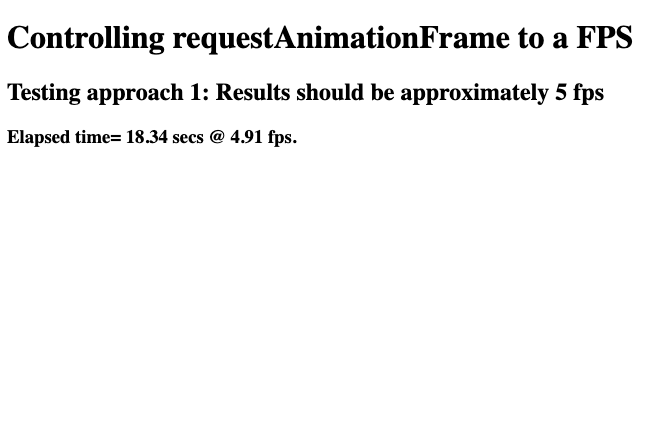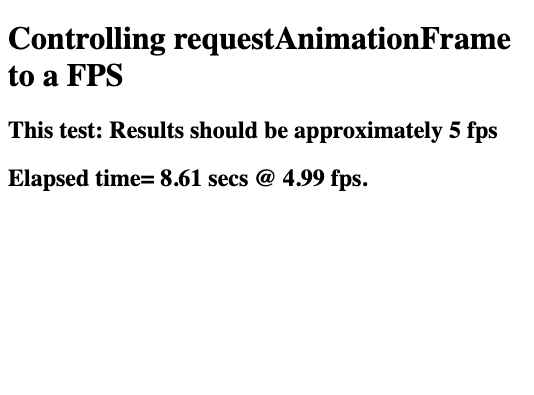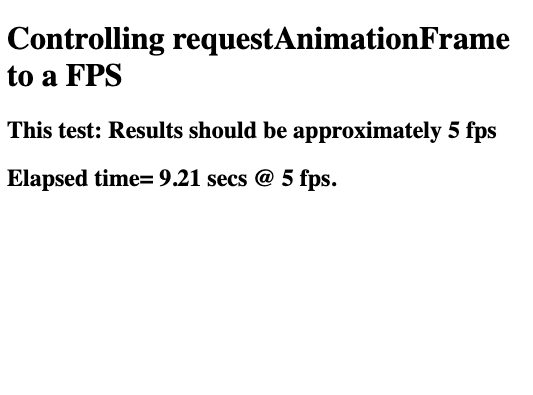# How to control fps with requestAnimationFrame?

Introduction:
It is important to control the frames per second limit, especially, when developing games where the animated objects should not exceed particular frames per second limit. The `requestAnimationFrame()` is used for simply repainting the screen — it is not a timer or a loop function.

requestAnimationFrame(callback):
Used to update or repaint the screen using the callback function specified.

Whenever a function call is made to `requestAnimationFrame()` the screen/frame is repainted according to the update code written by the developer, which makes it a suitable option for controlling the frame rate. There are two ways of controlling the fps with `requestAnimationFrame()`. These are discussed as follows.

• Using `setTimeout` Function:
This is a quick and easy approach to controlling the fps. The `setTimeout()` function has the following declaration:

setTimeout(function, milliseconds) :
Can be used to execute a function after waiting for the specified number of seconds.

The code to control fps using `setTimeout()` is given below, the `requestAnimationFrame()` is passed as a function to `setTimeout()` for regularly updating the screen at the specified fps.

 `<``h1``>Controlling requestAnimationFrame to a FPS ` `<``h2``>Testing approach 1:  ` `         ``Results should be approximately 5 fps ` `<``h3` `id``=``"results"``>Results: ` `<``canvas` `id``=``"canvas"` `width``=``"300"` `height``=``"300"``> ` ` ` ` `  `<``script``> ` `    ``var frameCount = 0; ` `    ``var \$results = \$("#results"); ` `    ``var fps, fpsInterval, startTime, now, then, elapsed; ` ` `  `    ``startAnimating(5); ` ` `  `    ``function startAnimating(fps) { ` `        ``fpsInterval = 1000 / fps; ` `        ``then = Date.now(); ` `        ``startTime = then; ` `        ``console.log(startTime); ` `        ``animate(); ` `    ``} ` ` `  `    ``function animate() { ` `        ``setTimeout(function () { ` `      ``// requestAnimationFrame() is called  ` `      ``// with animate() callback ` `      ``// to update content at specified fps ` `            ``requestAnimationFrame(animate); ` ` `  `            ``// ... Code for Animating the Objects ... ` `            ``now = Date.now(); ` `            ``var sinceStart = now - startTime; ` `            ``var currentFps = ` `  ``Math.round((1000 / (sinceStart / ++frameCount)) * 100) / 100; ` `   ``\$results.text("Elapsed time= "  ` `         ``+ Math.round((sinceStart / 1000) * 100) / 100 + ` `         ``" secs @ " + currentFps + " fps."); ` `        ``}, fpsInterval); ` `    ``} ` ` `

Output:
The two images show the fps fluctuating around 5 fps, as assumed by the above code.

• FPS is 4.9, after 5.31 seconds have elapsed:
•• FPS is 4.91, after 18.34 seconds have elapsed:
•The above code is simply, calling the `setTimeout()` function at the interval rate specified by the fps. Each time `setTimeout()` is called, the `requestAnimationFrame()` is executed and the screen is repainted or updated. All this occurs at the fps specified, as determined by the developer.

• A More Optimized Approach:
Most browsers cannot optimize the `setTimeout()` function, so to optimize the process of controlling fps simple calculation can be used. This can be easily done by keeping track of time when the frame was updated last. If the change in time (`current time — previous time`) exceeds the update interval, then update the frame/screen.

To do so, keep track of the current_time and the previous_time. Each time we get:

```current_time - previous_time > update_interval
```

the frame is updated and repainted onto the screen. The code for this is as follows:

 `<``h1``>Controlling requestAnimationFrame to a FPS ` `<``h2``>This test: Results should be approximately 5 fps ` `<``h2` `id``=``"results"``>Results: ` `<``canvas` `id``=``"canvas"` `width``=``"300"` `height``=``"300"``> ` ` `  `<``script``> ` `    ``var frameCount = 0; ` `    ``var \$results = \$("#results"); ` `    ``var fps, fpsInterval, startTime, now, then, elapsed; ` ` `  `    ``startAnimating(5); ` ` `  `    ``function startAnimating(fps) { ` `        ``fpsInterval = 1000 / fps; ` `        ``then = Date.now(); ` `        ``startTime = then; ` `        ``console.log(startTime); ` `        ``animate(); ` `    ``} ` ` `  `    ``function animate() { ` `        ``// request another frame ` ` `  `        ``requestAnimationFrame(animate); ` ` `  `        ``// calc elapsed time since the last loop ` ` `  `        ``now = Date.now(); ` `        ``elapsed = now - then; ` ` `  `        ``// if enough time has elapsed, draw the next frame ` ` `  `        ``if (elapsed > fpsInterval) { ` `            ``then = now - (elapsed % fpsInterval); ` ` `  `        ``// draw animating objects here... ` ` `  `        ``// below code is used for testing, whether ` `       ``// the frame is animating at the specified fps ` ` `  `            ``var sinceStart = now - startTime; ` `            ``var currentFps =  ` `Math.round((1000 / (sinceStart / ++frameCount)) * 100) / 100; ` `            ``\$results.text("Elapsed time= "  ` `             ``+ Math.round((sinceStart / 1000) * 100) / 100  ` `             ``+ " secs @ " + currentFps + " fps."); ` `        ``} ` `    ``} ` ` `

Output:
The two images show the fps fluctuating around 5 fps, as assumed by the above code.

• FPS is 4.99, after 8.61seconds have elapsed:
•• FPS is 5.00, after 9.21 seconds have elapsed:
•In the above code, two variable `current_time` and `previous_time` are used to keep track of the time elapsed since the last update. Whenever the condition is satisfied (change in time is greater than interval time), the frame is updated to animate the objects.My Personal Notes arrow_drop_upCheck out this Author's contributed articles.

If you like GeeksforGeeks and would like to contribute, you can also write an article using contribute.geeksforgeeks.org or mail your article to contribute@geeksforgeeks.org. See your article appearing on the GeeksforGeeks main page and help other Geeks.

Please Improve this article if you find anything incorrect by clicking on the "Improve Article" button below.

Article Tags :

Be the First to upvote.

Please write to us at contribute@geeksforgeeks.org to report any issue with the above content.